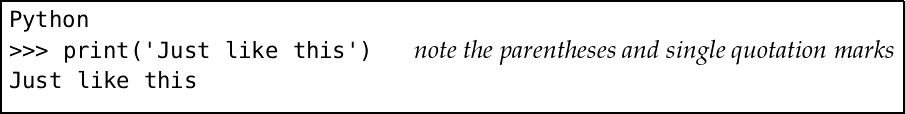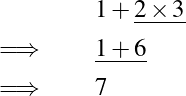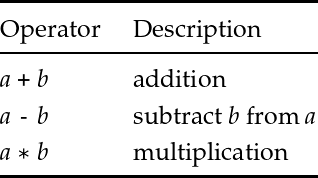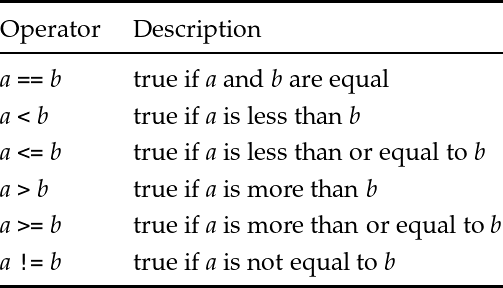Back to Contents

# 1. Starting Off

We will cover a fair amount of material in this chapter and its questions, since we will need a solid base on which to build. You should read this with a computer running Python in front of you.

### Expressions and statements

A computer program written in Python is built from statements and expressions. Each statement performs some action. For example, the built-in `print` statement writes to the screen:Each expression performs some calculation, yielding a value. For example, we can calculate the result of a simple mathematical expression using whole numbers (or integers):

``````Python
>>> 1 + 2 * 3
7``````

When Python has calculated the result of this expression, it prints it to the screen, even though we have not used `print`. All of our programs will be built from such statements and expressions.

The single quotation marks in our `print` statement indicate that what we are printing is a string – a sequence of letters or other symbols. If the string is to contain a single quotation mark, we must use the double quotation mark key instead:

``````Python
>>> print('Can't use single quotation marks here!')
File "<stdin>", line 1
print('Can't use single quotation marks here!')
^
SyntaxError: invalid syntax

>>> print("Can't use single quotation marks here!")
Can't use single quotation marks here!``````

Note that this is a different key on the keyboard – we are not typing two single quotation marks – it is `"` not `’ ’`. We can print numbers too, of course:

``````Python
>>> print(12)
12``````

Note that `12` and `’12’` are different things: one is the whole number (or integer) 12, and one is the string consisting of the two symbols `1` and `2`. Notice also the difference between an expression which is just a string, and the statement which is the act of printing a string:

``````Python
>>> 'Just like this'
'Just like this'
>>> print('Just like this')
Just like this``````

### Numbers

We have seen how to do mathematical calculations with our numbers, of course:

``````Python
>>> 1 + 2 * 3
7``````

Even quite large calculations:

``````Python
>>> 1000000000 + 2000000000 * 3000000000
6000000001000000000``````

Using the `_` underscore key to split up the numbers is optional, but helps with readability:

``````Python
>>> 1_000_000_000 + 2_000_000_000 * 3_000_000_000
6000000001000000000``````

Python reduces the mathematical expression `1 + 2 * 3` to the value `7` and then prints it to the screen. This expression contains the operators `+` and `*` and their operands `1`, `2`, and `3`.

How does Python know how to calculate `1 + 2 * 3`? Following known rules, just like we would. We know that the multiplication here should be done before the addition, and so does Python. So the calculation goes like this:The piece being processed at each stage is underlined. We say that the multiplication operator has higher precedence than the addition operator. Here are some of Python’s operators for arithmetic:In addition to our rule about `*` being performed before `+` and `-`, we also need a rule to say what is meant by `9 - 4 + 1`. Is it `(9 - 4) + 1` which is 6, or `9 - (4 + 1)` which is 4? As with normal arithmetic, it is the former in Python:

``````Python
>>> 9 - 4 + 1
6``````

This is known as the associativity of the operators.

### Truth and falsity

Of course, there are many more things than just numbers. Sometimes, instead of numbers, we would like to talk about truth: either something is true or it is not. For this we use boolean values, named after the English mathematician George Boole (1815–1864) who pioneered their use. There are just two booleans:

`True`
`False`

How can we use these? One way is to use one of the comparison operators, which are used for comparing values to one another:

``````Python
>>> 99 > 100
False
>>> 4 + 3 + 2 + 1 == 10
True``````

It is most important not to confuse `==` with `=` as the single `=` symbol means something else in Python. Here are the comparison operators:There are two operators for combining boolean values (for instance, those resulting from using the comparison operators). The expression a `and` b evaluates to `True` only if expressions a and b both evaluate to `True`. The expression a `or` b evaluates to `True` if a evaluates to `True` or b evaluates to `True`, or both do. Here are some examples of these operators in use:

``````Python
>>> 1 == 1 and 10 > 9
True
>>> 1 == 1 or 9 > 10
True``````

In each case, the expression a will be tested first – the second may not need to be tested at all. The `and` operator is performed before `or`, so a `and` b `or` c is the same as (a `and` b) `or` c. The expression `not` a gives `True` if a is `False` and vice versa:

``````Python
>>> not 1 == 1
False
>>> 1 == 2 or not 9 > 10
True``````

The comparison operators have a higher precedence than the so-called logical operators: so, for example, writing `not 1 == 1` is the same as writing `not (1 == 1)` rather than `(not 1) == 1`.

### The types of things

In this chapter we have seen three types of data: strings, integers and booleans. We can ask Python to tell us the type of a value or expression:

``````Python
>>> type('Hello!')
<class 'str'>
>>> type(25)
<class 'int'>
>>> type(1 + 2 * 3)
<class 'int'>
>>> type(False)
<class 'bool'>``````

Here, `’str’` indicates strings, `’bool’` booleans, and `’int’` integers.

## Common problems

When Python does not recognise what we type in as a valid program, an error message is shown instead of an answer. You will come across this many times when experimenting with your first Python programs, and part of learning to program is learning to recognise and fix these mistakes. For example, if we miss the quotation mark from the end of a string, we see this:

``````Python
>>> print('A string without a proper end)
File "<stdin>", line 1
print('A string without a proper end)
^
SyntaxError: EOL while scanning string literal``````

Such error messages are not always easy to understand. What is `EOL`? What is a `literal`? What is `<stdin>`? Nevertheless, you will become used to such messages, and how to fix your programs. In the next example, we try to compare a number to a string:

``````Python
>>> 1 < '2'
Traceback (most recent call last):
File "<stdin>", line 1, in <module>
TypeError: '<' not supported between instances of 'int' and 'str'``````

In this case the error message is a little easier to understand. Another common situation is missing out a closing parenthesis. In this case, Python does not know we have finished typing, even when we press Enter.

``````Python
>>> 2 * (3 + 4 + 5
...
...
...
... ``````

To get out of this situation, we can type Ctrl-C, to let Python know we wish to discard the statement and try again:

``````Python
>>> 2 * (3 + 4 + 5
...
...
...
...
KeyboardInterrupt``````

Or, if possible, we can finish the expression properly:

``````Python
>>> 2 * (3 + 4 + 5
...
...
...
...)
24``````

## Summary

We have learned how to interact with Python by typing statements and reading the answers. We have learned about three types of data: strings, whole numbers, and booleans. We have seen how to perform arithmetic on numbers, and how to test things for equality with one another, using operators and operands. We have learned about boolean operators too. Finally, we have learned how to ask Python to tell us the type of something.

In the next chapter, we will move on to more substantial programs. Meanwhile, there are some questions to try. Answers and hints are at the back of the book.

## Questions

1. What sorts of thing do the following expressions represent and to what do they evaluate, and why? See if you can work them out without the computer to begin with.

`17`

`1 + 2 * 3 + 4`

`400 > 200`

`1 != 1`

`True or False`

`True and False`

`’%’`

2. A programmer writes `1+2 * 3+4`. What does this evaluate to? What advice would you give them?

3. Python has a modulus or remainder operator, which finds the remainder of dividing one number by another. It is written `%`. Consider the evaluations of the expressions `1 + 2 % 3`, `(1 + 2) % 3`, and `1 + (2 % 3)`. What can you conclude about the `+` and `%` operators?

4. What is the effect of the comparison operators like `<` and `>` on strings? For example, to what does `’bacon’ < ’eggs’` evaluate? What about `’Bacon’ < ’bacon’`? What is the effect of the comparison operators on the booleans `True` and `False`?

5. What (if anything) do the following statements print on the screen? Can you work out or guess what they will do before typing them in?

`1 + 2`

`’one’ + ’two’`

`1 + ’two’`

`3 * ’1’`

`’1’ * 3`

`print(’1’ * 3)`

`True + 1`

`print(f’One and two is {1 + 2} and that is all.’)`

(The last of these uses Python in a way we have not yet mentioned.)